Open in App
Not now

# Cross-Entropy Cost Functions used in Classification

• Difficulty Level : Hard
• Last Updated : 16 Oct, 2021

### A Brief Idea of Cost Functions

How does your teacher assess whether you have studied throughout the academic year or not? She takes a test at the end and grades your performance by cross-checking your answers against the desired answers. If you have managed to maintain your accuracy and have shot your scores over a certain benchmark, you have passed. If you haven’t(as unlikely as it is), you need to improve your accuracy and attempt again.  So in crude words, tests are used to analyze how well you have performed in class.

In machine learning lingo, a ‘cost function‘ is used to evaluate the performance of a model. An important question that might arise is, how can I assess how well my model is performing? Just like the teacher assesses your accuracy by verifying your answers against the desired answers, you assess the model’s accuracy by comparing the values predicted by the model with the actual values. The cost function quantifies the difference between the actual value and the predicted value and stores it as a single-valued real number.  The cost function can analogously be called the ‘loss function‘ if the error in a single training example only is considered. Note that these are applicable only in supervised machine learning algorithms that leverage optimization techniques. Since the cost function is the measure of how much our predicted values are deviating from the correct labelled values, it can be considered to be an inadequacy metric. Hence, all optimization techniques tend to strive to minimize it.

In this article, we shall be covering the cost functions predominantly used in classification models only.

## The Cross-Entropy Cost Function

### The Idea behind Shannon Entropies

The Entropy of a random variable X can be measured as the uncertainty in the variables’ possible outcomes. This means the more the certainty/probability, the lesser is the entropy.

The formula to calculate the entropy can be represented as:

(1)(2)Let us take a simple example.

You have 3 hampers and each of them contains 10 candies.

The first hamper has 3 Eclairs and 7 Alpenliebes.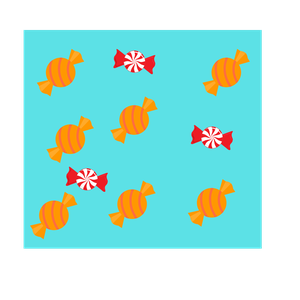Red=Eclairs, yellow=Alpenliebe

The second hamper has 5 Eclairs and 5 Alpenliebes.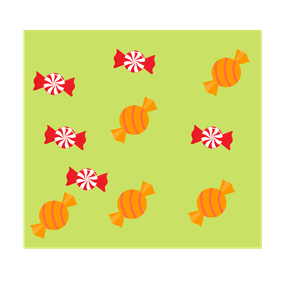The third hamper has 10 Eclairs and 0 Alpenliebes.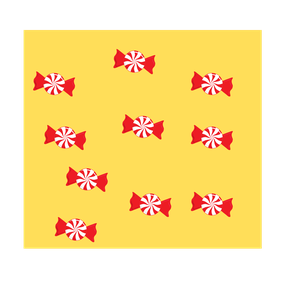Using the above equation, we can calculate the values of the entropies in each of the above cases.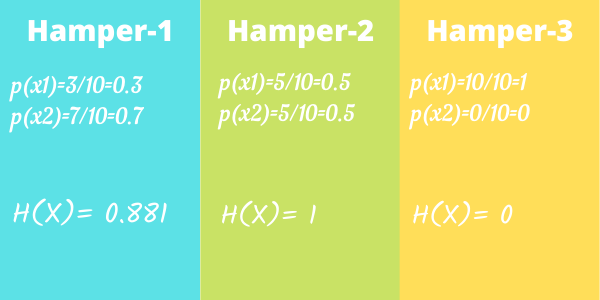You can now see that since hamper 2 has the highest degree of uncertainty, its entropy is the highest possible value, i.e 1. Also, since hamper 3 only has one kind of candies, there is 100% certainty that the candy drawn would be an Eclair. Therefore, there is no uncertainty and the entropy is 0.

### The Cost Function of Cross-Entropy

Now that you are familiar with entropy, let us delve further into the cost function of cross-entropy.

Let us take an example of a 3-class classification problem.  The model shall accept an image and distinguish whether the image can be classified as that of an apple’s, an orange’s or a mango’s. After processing, the model would provide an output in the form of a probability distribution. The predicted class would have the highest probability.

• Apple = [1,0,0]
• Orange = [0,1,0]
• Mango = [0,0,1]

This means that if the class correctly predicted by the model is, let’s say, apple. Then the predicted probability distribution of apple should tend towards the maximum probability distribution value, i.e, 1. If that is not the case, the weight of the model needs adjustment.

Let’s just say that the following logits were the predicted values: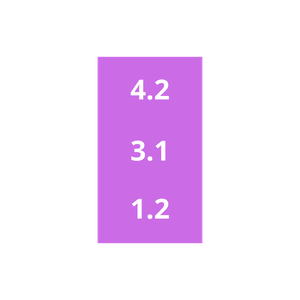Logits for apple, orange and mango respectively

These are the respective logit values for the input image being an apple, an orange and a mango. We can deploy a Softmax function to convert these logits into probabilities. The reason why we use softmax is that it is a continuously differentiable function. This makes it possible to calculate the derivative of the cost function for every weight in the neural network.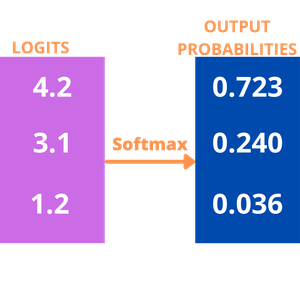Difference between the expected value and predicted value, ie 1 and 0.723= 0.277

Even though the probability for apple is not exactly 1, it is closer to 1 than all the other options are.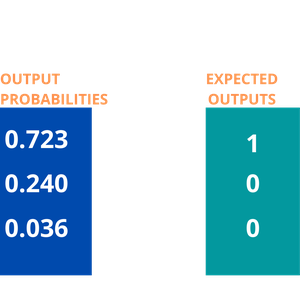After subsequent, successive iterative training, the model might improve its output probability considerably and reduce the loss. This is how cross-entropy can reduce the cost function and make the model more accurate. The formula used to predict the cost function is:

(3)## Multi-class Classification Cost Functions

Just like the aforementioned example, multi-class classification is the scenario wherein there are multiple classes, but the input fits in only 1 class. Fruit cannot practically be a mango and an orange both, right?

Let the model’s output highlight the probability distribution for ‘c’ classes for a fixed input ‘d‘.

(4)Also, let the actual probability distribution be

(5)Thus, the cross-entropy cost function can be represented as :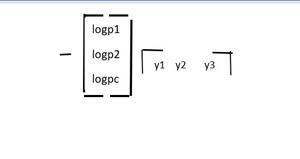Note that y3=yc for all ‘c’ termsNow, if we take the example of the probability distribution from the example on apples, oranges and mangoes and substitute the values in the formula, we get:

• p(Apple)=[0.723, 0.240, 0.036]
• y(Apple)=[1,0,0]

Cross-Entropy(y,P) loss = – (1*log(0.723) + 0*log(0.240)+0*log(0.036)) = 0.14

This is the value of the cross-entropy loss.

## Categorical Cross-Entropy

The error in classification for the complete model is given by the mean of cross-entropy for the complete training dataset. This is the categorical cross-entropy. Categorical cross-entropy is used when the actual-value labels are one-hot encoded. This means that only one ‘bit’ of data is true at a time, like [1,0,0], [0,1,0] or [0,0,1]. The categorical cross-entropy can be mathematically represented as:

Categorical Cross-Entropy = (Sum of Cross-Entropy for N data)/N

## Binary Cross-Entropy Cost Function

In Binary cross-entropy also, there is only one possible output. This output can have discrete values, either 0 or 1. For example, let an input of a particular fruit’s image be either that of an apple or that of an orange. Now, let us rewrite this sentence: A fruit is either an apple, or it is not an apple. There are only binary, true-false outputs possible.

Let us assume that the actual output is represented as a variable y

now, cross-entropy for a particular data ‘d’ can be simplified as

• Cross-entropy(d) = – y*log(p) when y = 1
• Cross-entropy(d) = – (1-y)*log(1-p) when y = 0

Problem implementation for this method is the same as those of multi-class cost functions. The difference is that only binary classes can be accepted.

## Sparse Categorical Cross-Entropy

In sparse categorical cross-entropy, truth labels are labelled with integral values. For example, if a 3-class problem is taken into consideration, the labels would be encoded as , , .

Note that binary cross-entropy cost-functions, categorical cross-entropy and sparse categorical cross-entropy are provided with the Keras API.

My Personal Notes arrow_drop_up